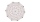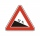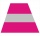# Rhombus

The rhombus has diagonal lengths of 4.2cm and 3.4cm. Calculate the length of the sides of the rhombus and its height

Result

a =  2.702 cm
h =  2.642 cm

#### Solution:

$u = 4.2 \ cm \ \\ v = 3.4 \ cm \ \\ \ \\ a = \sqrt{ (u/2)^2+(v/2)^2 } = \sqrt{ (4.2/2)^2+(3.4/2)^2 } \doteq 2.7019 = 2.702 \ \text { cm }$
$S = u \cdot \ v / 2 = 4.2 \cdot \ 3.4 / 2 = \dfrac{ 357 }{ 50 } = 7.14 \ cm^2 \ \\ \ \\ S = a \cdot \ h \ \\ h = S/a = 7.14/2.7019 = \dfrac{ 510 }{ 193 } \doteq 2.6425 = 2.642 \ \text { cm }$

Leave us a comment of this math problem and its solution (i.e. if it is still somewhat unclear...):Be the first to comment!#### Following knowledge from mathematics are needed to solve this word math problem:

Pythagorean theorem is the base for the right triangle calculator. Do you want to convert length units?

## Next similar math problems:

1. Cuboid face diagonalsThe lengths of the cuboid edges are in the ratio 1: 2: 3. Will the lengths of its diagonals be the same ratio? The cuboid has dimensions of 5 cm, 10 cm, and 15 cm. Calculate the size of the wall diagonals of this cuboid.
2. Wall and body diagonalsCalculate the lengths of the wall and body diagonals of the cuboid with edge dimensions of 0.5 m, 1 m, and 2 m
3. Body diagonalCalculate the volume of a cuboid whose body diagonal u is equal to 6.1 cm. Rectangular base has dimensions of 3.2 cm and 2.4 cm
4. Trapezoid MOThe rectangular trapezoid ABCD with right angle at point B, |AC| = 12, |CD| = 8, diagonals are perpendicular to each other. Calculate the perimeter and area of ​​the trapezoid.
5. Space diagonalThe space diagonal of a cube is 129.91 mm. Find the lateral area, surface area and the volume of the cube.
6. RectangleThe rectangle is 21 cm long and 38 cm wide. Determine the radius of the circle circumscribing rectangle.
7. Faces diagonalsIf the diagonals of a cuboid are x, y, and z (wall diagonals or three faces) respectively than find the volume of a cuboid. Solve for x=1.2, y=1.7, z=1.45
8. Medians in right triangleIt is given a right triangle, angle C is 90 degrees. I know it medians t1 = 8 cm and median t2 = 12 cm. .. How to calculate the length of the sides?
9. Right ΔA right triangle has the length of one leg 7 cm and length of the hypotenuse 25 cm. Calculate the height of the triangle.
10. Two chordsCalculate the length of chord AB and perpendicular chord BC to circle if AB is 4 cm from the center of the circle and BC 8 cm from the center of the circle.
11. GardenArea of a square garden is 6/4 of triangle garden with sides 56 m, 35 m, and 35 m. How many meters of fencing need to fence a square garden?
12. Axial sectionAxial section of the cone is an equilateral triangle with area 208 dm2. Calculate the volume of the cone.
13. Angles of elevationFrom points A and B on level ground, the angles of elevation of the top of a building are 25° and 37° respectively. If |AB| = 57m, calculate, to the nearest meter, the distances of the top of the building from A and B if they are both on the same side of t
14. Circle chordWhat is the length d of the chord circle of diameter 50 dm, if the distance from the center circle is 21 dm?
15. Uphill gardenI have a garden uphill, increasing from 0 to 4.5 m for a length of 25 m, how much is the climb in percent?
16. Secret treasureScouts have a tent in the shape of a regular quadrilateral pyramid with a side of the base 4 m and a height of 3 m. Determine the radius r (and height h) of the container so that they can hide the largest possible treasure.
17. Hole's anglesI am trying to find an angle. The top of the hole is .625” and the bottom of the hole is .532”. The hole depth is .250” what is the angle of the hole (and what is the formula)?